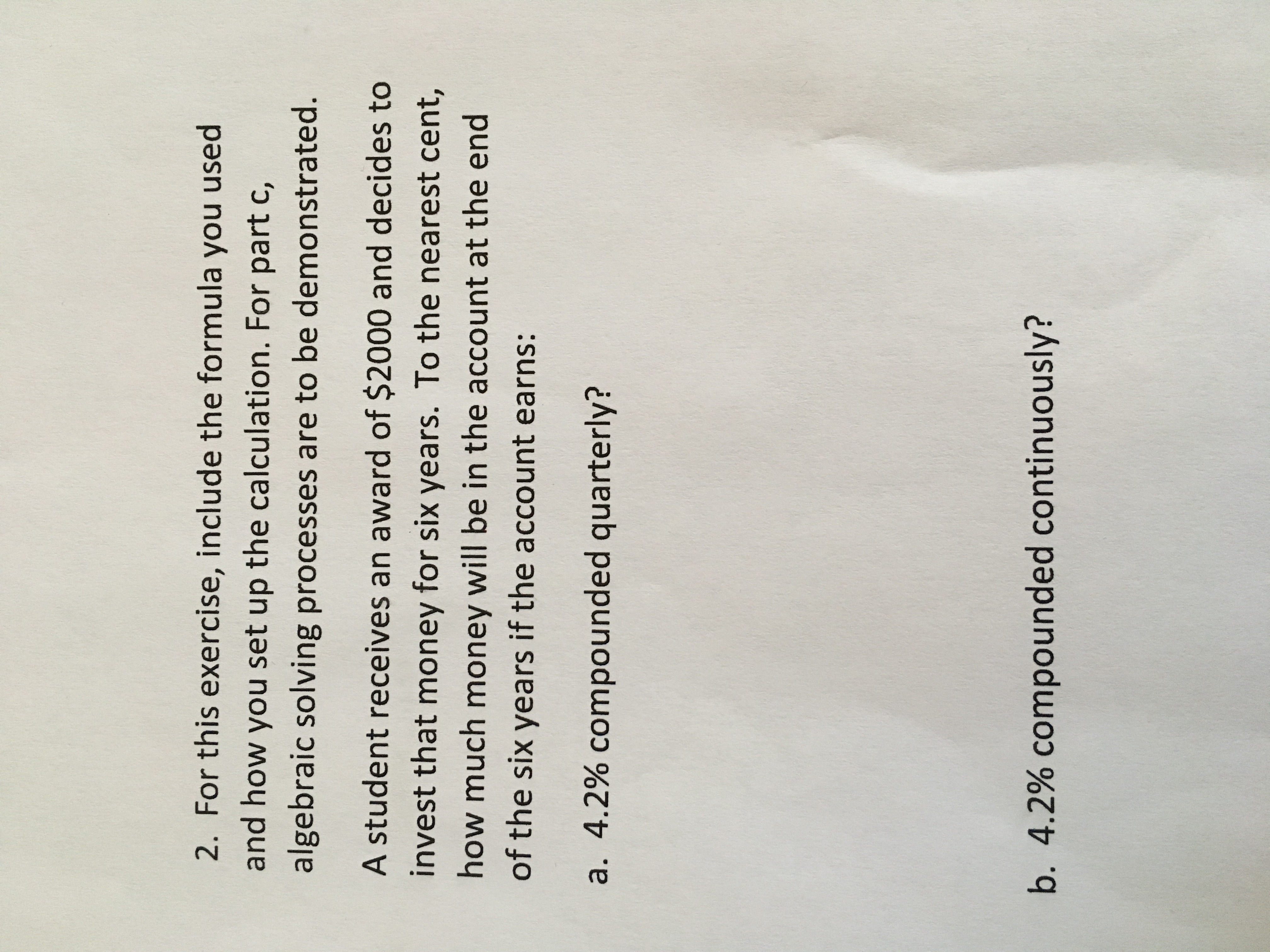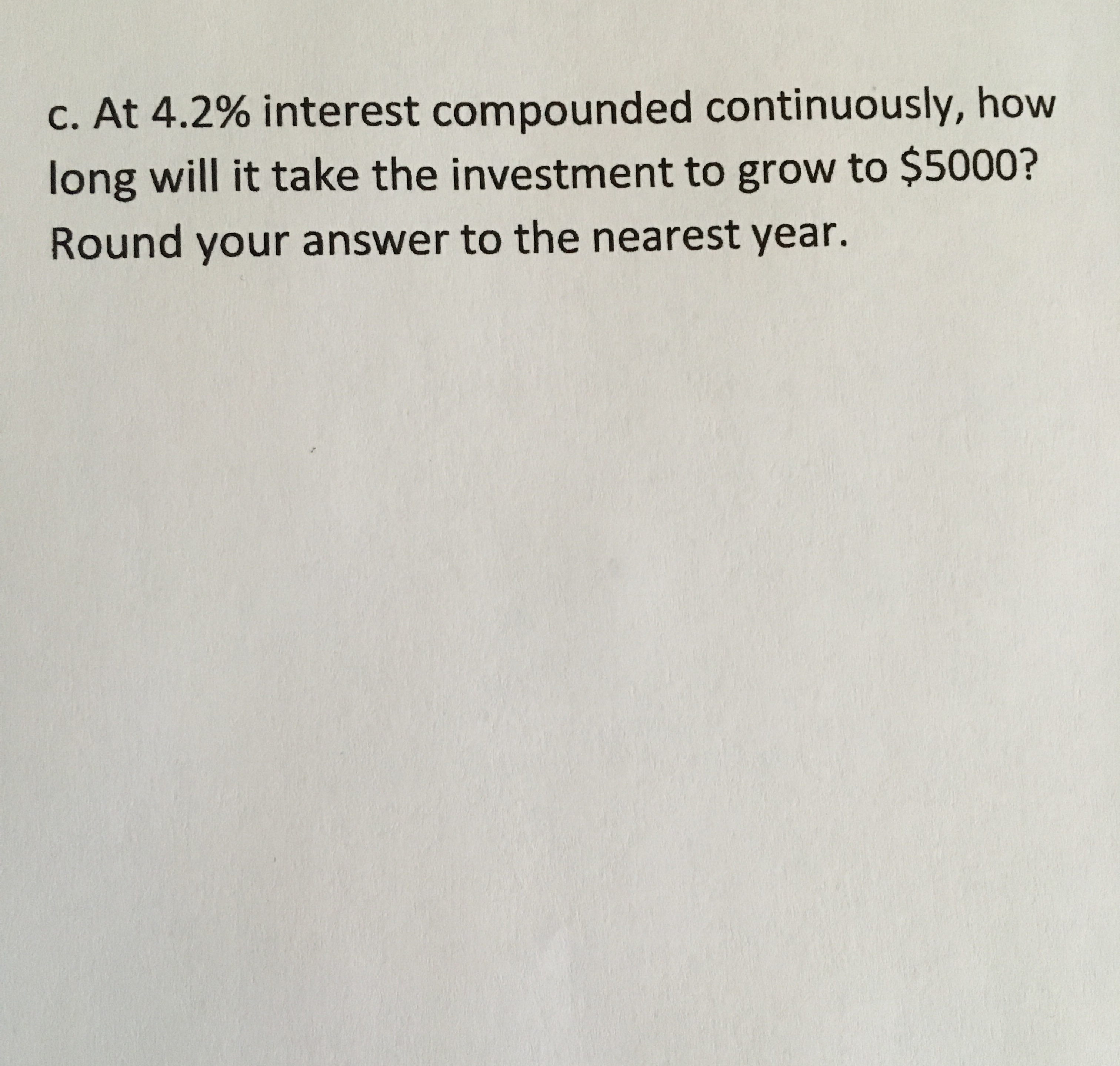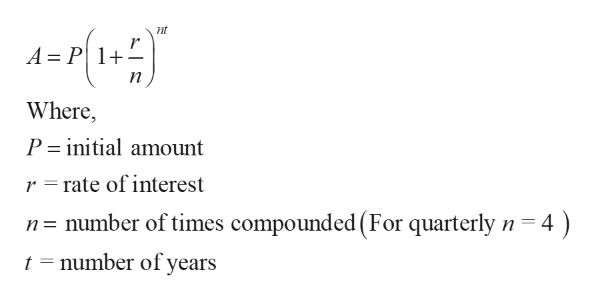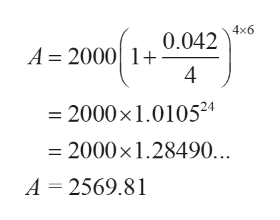# 2. For this exercise, include the formula you usedand how you set up the calculation. For part c,algebraic solving processes are to be demonstrated.A student receives an award of \$2000 and decides toinvest that money for six years. To the nearest cent,how much money will be in the account at the endof the six years if the account earns:a. 4.2% compounded quarterly?b. 4.2% compounded continuously? c. At 4.2% interest compounded continuously, howlong will it take the investment to grow to \$5000?Round your answer to the nearest year.

Question
138 viewshelp_outlineImage Transcriptionclose2. For this exercise, include the formula you used and how you set up the calculation. For part c, algebraic solving processes are to be demonstrated. A student receives an award of \$2000 and decides to invest that money for six years. To the nearest cent, how much money will be in the account at the end of the six years if the account earns: a. 4.2% compounded quarterly? b. 4.2% compounded continuously? fullscreenhelp_outlineImage Transcriptionclosec. At 4.2% interest compounded continuously, how long will it take the investment to grow to \$5000? Round your answer to the nearest year. fullscreen
check_circle

Step 1

From the given,

P = 2000

n = 6

r = 4.2% = 0.042

Step 2

a) Compounded quarterly at 4.2% interest

Compound interest formulahelp_outlineImage Transcriptionclosent A = P| 1+- Where, P = initial amount r =rate of interest n= number of times compounded (For quarterly n=4 ) t = number of years fullscreen
Step 3

Then,

...help_outlineImage Transcriptionclose4x6 0.042 A = 2000| 1+ 4 = 2000x1.0105²4 = 2000x1.28490... A = 2569.81 fullscreen

### Want to see the full answer?

See Solution

#### Want to see this answer and more?

Solutions are written by subject experts who are available 24/7. Questions are typically answered within 1 hour.*

See Solution
*Response times may vary by subject and question.
Tagged in

### Other